# GMAT Math : DSQ: Calculating the length of the side of a rectangle

## Example Questions

### Example Question #1 : Rectangles

Ronald is making a bookshelf with a rectangular base that will be two yards tall. What is the area of the base?

I) The distance around the base will beyards.

II) The smaller sides of the base are half the length of the longer sides.

Statement I is sufficient to answer the question, but Statement II is not sufficient to answer the question.

Either statement alone is sufficient to answer the question.

Both statements together are needed to answer the question.

Statement II is sufficient to answer the question, but Statement I is not sufficient to answer the question.

Both statements together are needed to answer the question.

Explanation:

To find the area we need the length and width of the rectangle. We can use II together with I to make an equation for perimeter with only one unknown.

So we need both to solve.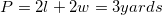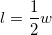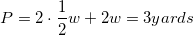Solve for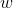and then go back to findand then with that you can find the area of the base and you are finished.

### Example Question #2 : Rectangles

Find a possible width of rectangle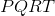.

I)has a perimeter of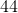fathoms.

II)has a diagonal length offathoms.

Statement I is sufficient to answer the question, but statement II is not sufficient to answer the question.

Both statements are needed to answer the question.

Statement II is sufficient to answer the question, but statement I is not sufficient to answer the question.

Either statement is sufficient to answer the question.

Both statements are needed to answer the question.

Explanation:

When asked to find the width of a rectangle we will need to use both statemests together.

For Statement I) we can use the perimeter formula.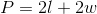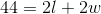Now, for Statement II) we will use the length of the diagonal along with the Pythagorean Theorem.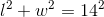From here you can solve the perimeter equation in terms of either l or w. Then you can use substitution into the Pythagorean Theorem to solve for a possible width.

### Example Question #3 : Rectangles

Find the length of the side of a rectangle with a width three times the length.

1. The area of the rectangle is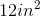.
2. The perimeter of the rectangle is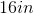Both statements taken together are sufficient to answer the question, but neither statement alone is sufficient.

Statement 1 alone is sufficient, but statement 2 alone is not sufficient to answer the question.

Statements 1 and 2 are not sufficient, and additional data is needed to answer the question.

Statement 2 alone is sufficient, but statement 1 alone is not sufficient to answer the question.

Each statement alone is sufficient to answer the question.

Each statement alone is sufficient to answer the question.

Explanation:

Statement 1: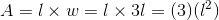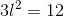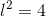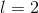Statement 1 is sufficient to answer the question

Statement 2: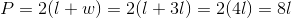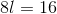Statement 2 is also sufficient to answer the question

### Example Question #4 : Rectangles

A rectangle has a width measuring twice the length. Find the length.

1. The rectangle has a perimeter of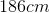.
2. The rectangle's area is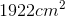.

Statements 1 and 2 are not sufficient, and additional data is needed to answer the question.

Both statements taken together are sufficient to answer the question, but neither statement alone is sufficient.

Statement 2 alone is sufficient, but statement 1 alone is not sufficient to answer the question.

Each statement alone is sufficient to answer the question.

Statement 1 alone is sufficient, but statement 2 alone is not sufficient to answer the question.

Each statement alone is sufficient to answer the question.

Explanation:

Statement 1:

Recall the formula to find the perimeter of a rectangle. Substitute in the given information and solve.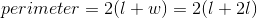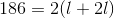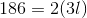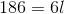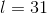Statement 2:

Recall the formula for the area of a rectangle. Substitute in the given information and solve.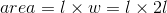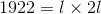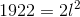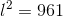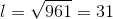Each statement alone is sufficient to answer the question.

### Example Question #5 : Rectangles

Given parallelogramwith diagonal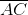. Is this parallelogram a rectangle?

1)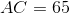2)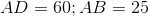BOTH statements TOGETHER are sufficient to answer the question, but NEITHER statement ALONE is sufficient to answer the question.

Statement 2 ALONE is sufficient to answer the question, but Statement 1 ALONE is NOT sufficient to answer the question.

EITHER statement ALONE is sufficient to answer the question.

Statement 1 ALONE is sufficient to answer the question, but Statement 2 ALONE is NOT sufficient to answer the question.

BOTH statements TOGETHER are insufficient to answer the question.

BOTH statements TOGETHER are sufficient to answer the question, but NEITHER statement ALONE is sufficient to answer the question.

Explanation:

The length of one diagonal alone does not prove the parallelogram to be a rectangle, nor do the lengths of the sides.

Suppose we know all of these lengths, though. Sinceis a parallelogram, if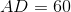, then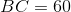The sides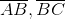and diagonalform a triangle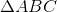with sidelengths 25, 60, and 65. The parallelogram is a rectangle if and only if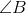is a right angle; therefore, we must determine whether the conditions of the Pythagorean Theorem hold: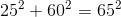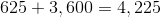This is true;is a right angle andis a rectangle.

Therefore, both statements together are sufficient to answer the question, but neither statement alone is sufficient to answer the question.

Tired of practice problems?

Try live online GMAT prep today.